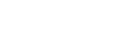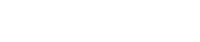AP Calculus Review: Derivatives of Inverse Functions

One of the trickiest topics on the AP Calculus AB/BC exam is the concept of inverse functions and their derivatives. In this review article, we’ll see how a powerful theorem can be used to find the derivatives of inverse functions. Then we’ll talk about the more common inverses and their derivatives.

What are Inverse Functions?

Basically, an inverse function is a function that “reverses” what the original function did.

For example, consider f(x) = 3x – 6. What does f do to its input x? Using correct order of operations, f has the following effect:

1. First multiply by 3.
2. Then subtract 6 from the result.

So, in order to reverse what f does, we have to follow the steps backwards. This is very much like reversing your driving directions to return home from an unfamiliar place.

1. First add 6 (to undo the subtraction).
2. Then divide by 3 (to undo the multiplication).

Therefore, the inverse function, which we’ll call g(x) for right now, has the formula,

g(x) = (x + 6)/3

The notation for the inverse function of f is f -1. So we could write:

f -1(x) = (x + 6)/3

Our purpose here is not to be able to solve to find inverse functions in all cases. In fact, the main theorem for finding their derivatives does not require solving for f -1(x) explicitly.

Finding the Derivative of an Inverse Function

Computing the derivative of an inverse function is not too much more difficult than computing derivatives in general.

First, here’s a quick review of the basic derivative rules: Calculus Review: Derivative Rules.

The Main Theorem for Inverses

Suppose that f is a function that has a well-defined inverse f -1, and suppose that (a, b) is a point on the graph of y = f(x). ThenHowever, you might see a different version of this rule. Another way to say that (a, b) is a point on the graph of y = f(x) is to say that b = f(a). Moreover, by properties of the inverse, then we can say that a = f -1(b).

Finally, replacing b by x, we discover the second version of the Main Theorem:The two versions are useful in different contexts, which we shall see in the examples.

It’s also good to know that the condition of “having a well-defined inverse” is satisfied whenever the function is one-to-one.

Example 1

Suppose that g(x) is the inverse function for f(x) = 3x5 + 6x3 + 4. Find the value of g '(13).

Solution

It won’t do us any good to try to solve for the inverse function algebraically. This polynomial is just too complicated for that. Instead, we must rely on the Main Theorem.

First, find the derivative of the original function.

f '(x) = 15x4 + 18x2

The other piece of the puzzle is the value to plug in. Identify b = 13 from the problem statement. But don’t plug 13 into anything! Instead, the formula requires a value a such that f(a) = b; that is, f(a) = 13.

So it looks like we might have to solve:

3x5 + 6x3 + 4 = 13

However, that polynomial is still way to difficult to solve algebraically. Fortunately, it doesn’t take long to guess and check a value for x that would make this equation true.

Just look at the coefficients: they add up to 13… Thus, if we just plugged in x = 1, then we’d get the correct result.

3(1)5 + 6(1)3 + 4 = 3 + 6 + 4 = 13

This means we should use a = 1 in the formula for the derivative.

Finally, put it all together using the formula from the Main Theorem:

g '(b) = 1/f '(a)

g '(13) = 1/f '(1) = 1/(15(1)4 + 18(1)2) = 1/33.

Common Inverses and Their Derivatives

Some functions are so important that their inverses get special names. We’ll take a look at two such cases:

• Logarithms, and
• Inverse trig functions

Logarithmic Functions

Logarithms are nothing more than the inverses of exponentiation. For a quick review, check out: AP Calculus Review: Properties of Exponents and Logarithms.

For our purposes in this article, it suffices to understand the following inverse relationship.

• If f(x) = ax, then f -1(x) = loga x

So in particular, when the base is e, the natural base, then we get a relationship between ex and the natural logarithm, ln x.

• If f(x) = ex, then f -1(x) = ln x

Let’ use the Main Theorem to prove that the derivative of ln x is indeed equal to 1/x. Remember, if f(x) = ex, then f '(x) = ex as well.You could do a similar thing to calculate the derivative of loga x. But it’s easier just to memorize the end result.

Here are the derivatives of the logarithmic functions:Trigonometric Functions

Each of the six trig functions has its own inverse function. (Each of them requires a certain restriction on the domain of the original function to ensure that it’s one-to-one. But we won’t get into the technical details here.)

The inverse for sin x is either written    sin -1 x   or   arcsin x.

Similar notation applies for the other five.

You can use the Main Theorem for inverse functions to work out their derivatives, but it’s better just to have these memorized.Derivatives of inverse trigonometric functions

Example 2

Find the slope of the tangent line to y = arctan 5x at x = 1/5.

Solution

We know that arctan x is the inverse function for tan x, but instead of using the Main Theorem, let’s just assume we have the derivative memorized already. (You can cheat and look at the above table for now… I won’t tell anyone.)

When you take the derivative, keep in mind that there will be a Chain Rule, with inside function u = 5x and outside function arctan u.Then plug in x = 1/5 to compute the slope.Improve your SAT or ACT score, guaranteed. Start your 1 Week Free Trial of Magoosh SAT Prep or your 1 Week Free Trial of Magoosh ACT Prep today!Author

•Shaun earned his Ph. D. in mathematics from The Ohio State University in 2008 (Go Bucks!!). He received his BA in Mathematics with a minor in computer science from Oberlin College in 2002. In addition, Shaun earned a B. Mus. from the Oberlin Conservatory in the same year, with a major in music composition. Shaun still loves music -- almost as much as math! -- and he (thinks he) can play piano, guitar, and bass. Shaun has taught and tutored students in mathematics for about a decade, and hopes his experience can help you to succeed!